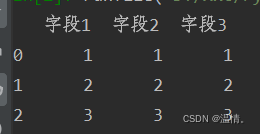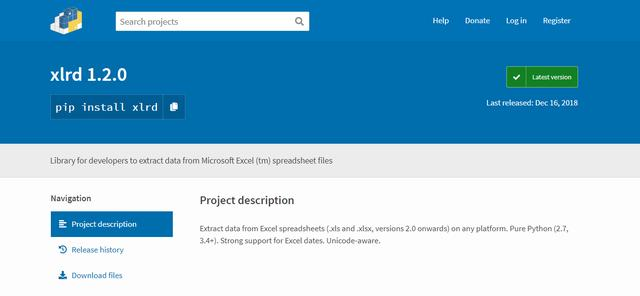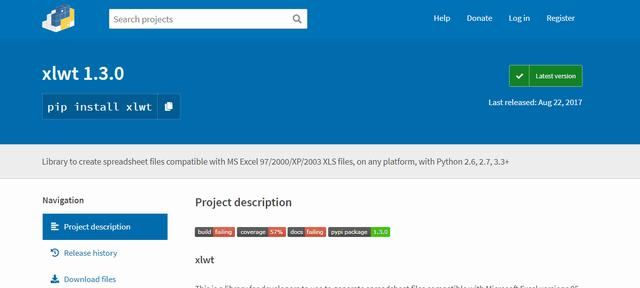• python导入excel数据到mysql
千次阅读
2018-05-22 13:38:38

# python导入excel数据到mysql

• 使用多线程，目前大概一分钟写入1w条

1. 环境介绍
• windows10-x64
• python3.6.5-x64
• Excel2016
• MySql5.7.18
2. 需要用到的资源
3. 源码及说明

• 源码
import pymysql # 操作mysql的模块
import openpyxl # xlsx格式对应的操作模块
import time
import math
from datetime import datetime

successList = [] # 储存每个线程成功的数目，用于统计

conn = pymysql.connect(host="192.168.0.243",port=3306,user="root",passwd="root",db="mydb",charset="utf8")
cur = conn.cursor() # 获取游标

num = 0
for row in rows:

itemNo = row.value if row.value != None else 111
itemName = row.value.replace("'","") if row.value != None else ""
itemName = itemName.replace("\\", "|")
pym = row.value.replace("'","") if row.value != None else ""
pym = pym.replace("\\", "|")
itemSize = row.value.replace("'","") if row.value != None else ""
itemSize = itemSize.replace("\\", "|")
unitNo = row.value.replace("'","") if row.value != None else ""
unitNo = unitNo.replace("\\", "|")
productArea = row.value.replace("'","") if row.value != None else ""
productArea = productArea.replace("\\", "|")

args = (itemNo,itemName,pym,itemSize,unitNo,productArea)
print(args)
try:
sql = r'''
insert into bar_code_dcm1 (itemNo,itemName,pym,itemSize,unitNo,productArea)
values
(%s,'%s','%s','%s','%s','%s')
''' % args
# print(sql, "\r\n----------------------------------------------------------")
cur.execute(sql)
conn.commit()
num = num +1
except Exception as e:
print(Exception, e,"SQL：%s " % sql)
else:
pass
finally:
pass

if num % 1000 == 0:
print("---当前线程已导入：", num," 条   %s" % time.strftime("%Y-%m-%d %H:%M:%S", time.localtime()) )
successList.append(num)
conn.close()
# time.sleep(1)

def excel2Mysql(excelFileName):

wb = openpyxl.load_workbook(excelFileName)  # 打开excel文件 ；16.5s
sheetList = wb.sheetnames# 获取工作簿所有工作表名

for sheetName in sheetList: # 遍历，每一个工作簿

sheetObj = wb.[sheetName] #获取工作簿对象
rows = sheetObj.iter_rows()

bigList = [] # 每个元素为一行excel表格内容
poolArgsList = [] # 每个元素为一万行excel表格内容,传递给线程池的集合

for row in rows:
if len(row.value) != 13: # 过滤掉不标准的条形码数据（标准数字条形码长度为13）
continue
else:
bigList.append(row)

cycle = math.ceil(len(bigList) / 10000)
for index in range(1,cycle+1):
thisList = bigList[(index-1)*10000:index*10000] # list切片
poolArgsList.append(thisList)

print("-*-数据读取，组装完毕-*-*-*开启 %d 个线程-*-*-*- \n\r" % 10)
pools.wait()

wb.close()

if __name__ == '__main__':
startTime = datetime.now()
excelFileName = "C:/Users/xusanduo/Desktop/excelData/50-55w.xlsx"
print("[  %s  ] [ 开始导入 %s " % ( time.strftime("%Y-%m-%d %H:%M:%S", time.localtime() ), excelFileName),"文件 ]" )
excel2Mysql(excelFileName)
endTime = datetime.now()
print( "[  %s  ] %s " % ( time.strftime("%Y-%m-%d %H:%M:%S", time.localtime() ) , "[ 导入完毕，总导入：" + str(sum(successList)) + "条 ]") , "[ 用时 %d 秒]" % (endTime-startTime).seconds )
• 关于源码的说明:
• 你可能需要安装pymysql ，openpyxl ，threadpool 模块，如果你没有的话，执行命令：pip install [module_name]
• 你可能需要修改读取的文件绝对路径
• 你可能需要修改mysql的连接参数mysql
更多相关内容
• import pandas as pd # 导入pandas模块 # 解决数据输出时列名不对齐的问题 pd.set_option('display.unicode.east_asian_width',True) df = pd.read_excel('data.xlsx') # 读取excel文件 print(df.head())

测试数据代码

import pandas as pd         # 导入pandas模块
# 解决数据输出时列名不对齐的问题
pd.set_option('display.unicode.east_asian_width',True)


输出结果pandas默认输出结果，会出现列不对齐或者多行多列显示不全的问题，使用set_option函数可以解决这两个问题。

 解决列名不对齐
通过将display.unicode.east_asian_width设置为TRUE，使列名对齐。例如：

pd.set_option('display.unicode.east_asian_width',True)


行列显示不全
通过将display.max_rows和display.max_columns修改为默认输出最大的行数和列数。例如：

pd.set_option('display.max_rows',1000)
pd.set_option('display.max_columns',1000)

展开全文• 一个简单的python程序带图形化界面用于读取excel将其中数据导入数据库
• 因为近期需要将excel导入到SQL Server，但是使用的是其他语言，闲来无事就尝试着用python进行导入，速度还是挺快的，1w多条数据，也只用了1s多，代码也比较简单，就不多解释了。 用到的库有xlrd(用来处理excel)，...
• 2、利用xlrd中的open_workbook函数读入excel文件，即可在python导入excel数据。一、安装xlrd模块到python官网下载http://pypi.python.org/pypi/xlrd模块安装，前提是已经安装了python 环境。二、使用介绍1、导入...

python将数据导入excel的方法：1、在python官网下载xlrd第三方库；2、利用xlrd中的open_workbook函数读入excel文件，即可在python中导入excel数据。一、安装xlrd模块

到python官网下载http://pypi.python.org/pypi/xlrd模块安装，前提是已经安装了python 环境。

二、使用介绍

1、导入模块import xlrd

2、打开Excel文件读取数据data = xlrd.open_workbook('excelFile.xls')

3、使用技巧获取一个工作表table = data.sheets() #通过索引顺序获取

table = data.sheet_by_index(0) #通过索引顺序获取

table = data.sheet_by_name(u'Sheet1')#通过名称获取获取整行和整列的值（数组）table.row_values(i)

table.col_values(i)获取行数和列数nrows = table.nrows

ncols = table.ncols循环行列表数据for i in range(nrows ):

print table.row_values(i)单元格cell_A1 = table.cell(0,0).value

cell_C4 = table.cell(2,3).value使用行列索引cell_A1 = table.row(0).value

cell_A2 = table.col(1).value简单的写入row = 0

col = 0

# 类型 0 empty,1 string, 2 number, 3 date, 4 boolean, 5 error

ctype = 1 value = '单元格的值'

xf = 0 # 扩展的格式化

table.put_cell(row, col, ctype, value, xf)

table.cell(0,0) #单元格的值'

table.cell(0,0).value #单元格的值'

三、Demo代码

Demo代码其实很简单，就是读取Excel数据。# -*- coding: utf-8 -*-

import xdrlib ,sys

import xlrd

def open_excel(file= 'file.xls'):

try:

data = xlrd.open_workbook(file)

return data

except Exception,e:

print str(e)

#根据索引获取Excel表格中的数据 参数:file：Excel文件路径 colnameindex：表头列名所在行的所以 ，by_index：表的索引

def excel_table_byindex(file= 'file.xls',colnameindex=0,by_index=0):

data = open_excel(file)

table = data.sheets()[by_index]

nrows = table.nrows #行数

ncols = table.ncols #列数

colnames = table.row_values(colnameindex) #某一行数据

list =[]

for rownum in range(1,nrows):

row = table.row_values(rownum)

if row:

app = {}

for i in range(len(colnames)):

app[colnames[i]] = row[i]

list.append(app)

return list

#根据名称获取Excel表格中的数据 参数:file：Excel文件路径 colnameindex：表头列名所在行的所以 ，by_name：Sheet1名称

def excel_table_byname(file= 'file.xls',colnameindex=0,by_name=u'Sheet1'):

data = open_excel(file)

table = data.sheet_by_name(by_name)

nrows = table.nrows #行数

colnames = table.row_values(colnameindex) #某一行数据

list =[]

for rownum in range(1,nrows):

row = table.row_values(rownum)

if row:

app = {}

for i in range(len(colnames)):

app[colnames[i]] = row[i]

list.append(app)

return list

def main():

tables = excel_table_byindex()

for row in tables:

print row

tables = excel_table_byname()

for row in tables:

print row

if __name__=="__main__":

main()

展开全文• 主要介绍了Python导入数值型Excel数据并生成矩阵操作，具有很好的参考价值，希望对大家有所帮助。一起跟随小编过来看看吧
• 原标题：如何将Excel导入Python之中呢？如何将Excel导入Python之中呢？...大概的流程是这样的：1、导入模块import xlrd2、打开Excel文件读取数据data = xlrd.open_workbook('excel.xls')3、获取一个工作...

原标题：如何将Excel导入Python之中呢？

如何将Excel导入Python之中呢？很简单！做法如下：

首先我们要读取excel要用到xlrd模块，官网安装先上官网安装。然后就可以跟着里面的例子稍微试一下就知道怎么用了。大概的流程是这样的：

1、导入模块

import xlrd

2、打开Excel文件读取数据

data = xlrd.open_workbook('excel.xls')

3、获取一个工作表

① table = data.sheets() #通过索引顺序获取

② table = data.sheet_by_index(0) #通过索引顺序获取

③ table = data.sheet_by_name(u'Sheet1')#通过名称获取

4、获取整行和整列的值（返回数组）

table.row_values(i)

table.col_values(i)

5、获取行数和列数

table.nrows

table.ncols

6、获取单元格

table.cell(0,0).value

table.cell(2,3).value

相对来说获取cell比较有用，相当于是给了一个二维数组，剩下的想怎么做都随着自己性子来。这全部归功于代码的简洁实用。若其中仍有若干小坑则花点时间自己琢磨下吧。以下列出的方法供人参考：

1、首先就是我的统计是根据姓名统计各个表中的信息的，但是调试发现不同的表中各个名字貌似不能够匹配，开始怀疑过编码问题，不过后来发现是因为 空格。因为在excel中输入的时候很可能会顺手在一些名字后面加上几个空格或是tab键，这样看起来没什么差别，但是程序处理的时候这就是两个完全 不同的串了。我的解决方法是给每个获取的字符串都加上strip（）处理一下。效果良好

2、还是字符串的匹配，在判断某个单元格中的字符串（中文）是否等于我所给出的的时候发现无法匹配，并且各种unicode也不太奏效，百度过一些解决 方案，但是都比较复杂或是没用。最后我采用了一个比较变通的方式：直接从excel中获取我想要的值再进行比较，效果是不错就是通用行不太好，个 呢不能问题还没解决。

二、写excel表

写excel表要用到xlwt模块，可到官网下载下载后大致的操作流程如下：

1、导入模块，复制代码代码 ：

import xlwt

2、创建workbook，就是excel，这里只需要保存下，然后复制代码：

workbook = xlwt.Workbook(encoding = 'ascii')

3、接着创建表，然后复制代码：

4、再往单元格内写入内容，复制代码代码：

worksheet.write(0, 0, label = 'Row 0, Column 0 Value')

5、最后保存，复制代码代码如下：

workbook.save('Excel_Workbook.xls')

以上便是小编对“如何将Excel导入Python之中呢？”的大致介绍，希望能有所帮助！返回搜狐，查看更多

责任编辑：

展开全文• python读写excel文件有很多种方法： 用xlrd和xlwt进行excel读写 用openpyxl进行excel读写 用pandas进行excel读写 本文使用xlrd读取excel文件（xls,sxls格式)，使用xlwt向excel写入数据 一、xlrd和xlwt的安装 安装...
• 主要介绍了使用pythonexcel数据导入数据库过程详解,文中通过示例代码介绍的非常详细，对大家的学习或者工作具有一定的参考学习价值,需要的朋友可以参考下
• PythonExcel的读写主要有:xlrd、xlwt、xlutils、openpyxl、xlsxwriter几种xlutils结合xlrd: 操作的是以xls后缀的excel，读取文件保留原格式：加：formatting_info=True常用方法：1、打开xls：open_workbook...
• 2、利用xlrd中的open_workbook函数读入excel文件，即可在python导入excel数据。一、安装xlrd模块到python官网下载http://pypi.python.org/pypi/xlrd模块安装，前提是已经安装了python 环境。二、使用介绍1、导入...
• 本文实例为大家分享了python处理excel绘制雷达图的具体代码，供大家参考，具体内容如下 python处理excel制成雷达图，利用工具plotly在线生成，事先要安装好xlrd组件 代码： import xlrd //事先要下载好xlrd组件 ...
• 使用python导入Excel作图 使用Excel数据作图： import pandas as pd import numpy as np import matplotlib.pyplot as plt from mpl_toolkits.axes_grid1.inset_locator import inset_axes from matplotlib.patches ...
• 使用python3导入excel文件到mysql。资源包含(excel文件，python源码，建表语句)，使用多线程，一分钟一万条的速度(无大字段)
• python pandas to_sql将excel数据导入到MySQL数据库用python pandas to_sql写了个脚本，向MySQL数据库导入excel数据，本地其实吧， 一分钟10W条数据不能算太快，10秒10W条还差不多。 可以研究一下线程+进程来处理， ...
• 根据需求建立数据库,建立了两个表，并保证了可以将数据存储到已有的数据库中，代码如下： import sqlite3 def createDataBase(): cn = sqlite3.connect('check.db') cn.execute('''CREATE TABLE IF NOT EXISTS TB_...
• 今天我们来学习数据导入，需要使用到Pandas模块。模块相当于是一个文件夹，它能够用于存放同个主题相关的Python代码段，包括定义函数、类和变量。例如将数据处理相关的函数都存放在一个模块里，将数据分析相关的函数...
• 本文是介绍自己如何将excel数据导入到Mysql中，以及中间有一个报错的解决方法，记录下自己的改正过程，嘻嘻嘻。 首先安装工具包，我用的jupyter notebook： !pip install mysqlclient # 导入需要的包 import pandas...mysql
• 前言公司原采用Excel表格方式记录着服务器资产信息，随着业务的增加，相应的硬件资产也增加，同时物理机虚拟化出多台虚拟机，存在表格管理杂乱、变更资产信息不能及时相互同步， 为了紧跟时代的步伐，老大搞了个基于...
• 本文实例讲述了Python导入oracle数据的方法。分享给大家供大家参考。具体如下： import cx_Oracle dns_tns=cx_Oracle.makedsn("192.168.0.288",1521,"skynet") print dns_tns con = cx_Oracle.connect('alibaba', '...
• 本文实例为大家分享了python操作EXCEL的实例源码，供大家参考，具体内容如下 读EXCEL的操作：把excel数据存储为字典类型 #coding=utf8 #导入excel的操作库 import xlrd class GenExceptData(object): def __...
• Python 导入 Excel 到数据库 ...本文将介绍如何基于Python3实现读取Excel数据，并导入到数据库。 本程序中会应用到两个包，可以通过pip安装 pip install openpyxl pyodbc 程序结构如下图所示 入口模块通过
• 在这段代码中，我从名为“emails”的excel表中导入了两个列表中的数据：a.列表1b.列表2处理这些数据并将其存储在最后两个列表中：a.最终清单1b.最终清单2现在我想将这两个最终列表中的数据存储在同一个excel中，我不...
• Python的一大应用就是数据分析了，而数据分析中，经常碰到需要处理Excel数据的情况。这里做一个Python处理Excel数据的总结，基本受用大部分情况。相信以后用Python处理Excel数据不再是难事儿！ xlwt库将数据导入...开发语言
• pythonExcel数据导入并转换成字典类型 1.导包 import xlrd class ExcelUtill(): def __init__(self,excelpath,sheetName="Sheet1"): self.data = xlrd.open_workbook(excelpath) self.table = self.data.sheet_...
• 主要用到的库有 xlrd和 pymysql, 注意pymysql不支持python3 篇幅有限，只针对主要操作进行说明 连接数据库 首先pymysql需要连接数据库，我这里连接的是本地数据库（数据库叫lds714610）。 conn = connect( ...mysql 数据库 编程语言
• python日记——Pandas之Excel绘图 利用pandas和pyplot进行数据可视化，绘图过程中使用到的excel文件如下：excel文件，提取码：falj 柱图的绘制 1、柱状图： import pandas as pd import matplotlib.pyplot as plt ......